21 生活中的设计模式：那些未完待续的设计模式

模板模式

Demo

from abc import ABCMeta, abstractmethod
# 引入ABCMeta和abstractmethod来定义抽象类和抽象方法

"阅读器视图"

def __init__(self):
self.__curPageNum = 1

def getPage(self, pageNum):
self.__curPageNum = pageNum
return "第" + str(pageNum) + "的内容"

def prePage(self):
content = self.getPage(self.__curPageNum - 1)
self.displayPage(content)

def nextPage(self):
content = self.getPage(self.__curPageNum + 1)
self.displayPage(content)

@abstractmethod
def displayPage(self, content):
"翻页效果"
pass

"左右平滑的视图"

def displayPage(self, content):
print("左右平滑:" + content)

"仿真翻页的视图"

def displayPage(self, content):
print("仿真翻页:" + content)

Define the skeleton of an algorithm in an operation, deferring some steps to client subclasses. Template Method lets subclasses redefine certain steps of an algorithm without changing the algorithm's structure.

代码框架

from abc import ABCMeta, abstractmethod
# 引入ABCMeta和abstractmethod来定义抽象类和抽象方法

class Template(metaclass=ABCMeta):
"模板类(抽象类)"

@abstractmethod
def stepOne(self):
pass

@abstractmethod
def stepTwo(self):
pass

@abstractmethod
def stepThree(self):
pass

def templateMethold(self):
"模板方法"
self.stepOne()
self.stepTwo()
self.stepThree()

class TemplateImplA(Template):
"模板实现类A"

def stepOne(self):
print("步骤一")

def stepTwo(self):
print("步骤二")

def stepThree(self):
print("步骤三")

class TemplateImplB(Template):
"模板实现类B"

def stepOne(self):
print("Step one")

def stepTwo(self):
print("Step two")

def stepThree(self):
print("Step three")

类图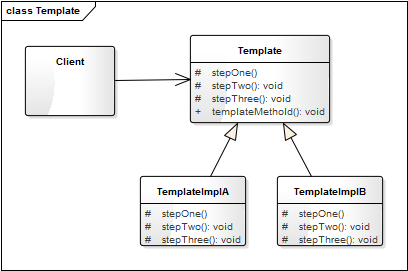Template 是一个模板类，用于定义模板方法（某种算法的框架），如示例中的 ReaderView。TemplateImplA 和 TemplateImplB 是模板类的具体子类，用于实现算法框架中的一些特定步骤。

应用场景

• 对一些复杂的算法进行分割，将其算法中固定不变的部分设计为模板方法和父类具体方法，而一些可以改变的细节由其子类来实现。即一次性实现一个算法的不变部分，并将可变的行为留给子类来实现。
• 各子类中公共的行为应被提取出来并集中到一个公共父类中以避免代码重复。
• 需要通过子类来决定父类算法中某个步骤是否执行，以实现子类对父类的反向控制。

桥接模式

类图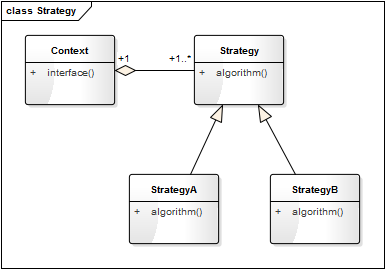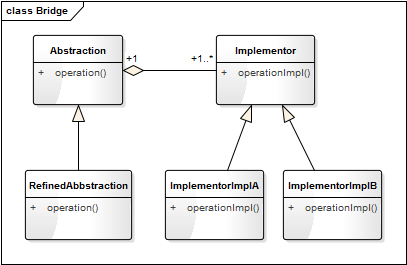桥接模式

Decouple an abstraction from its implementation so that the two can vary independently.

应用案例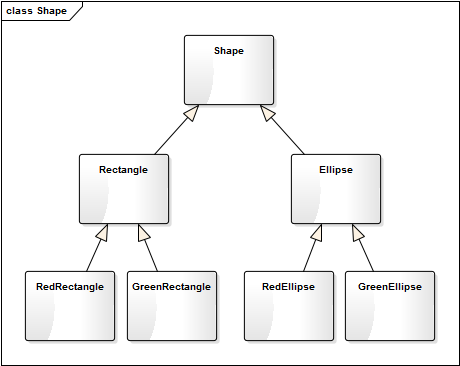from abc import ABCMeta, abstractmethod
# 引入ABCMeta和abstractmethod来定义抽象类和抽象方法

class Shape(metaclass=ABCMeta):
"形状"

def __init__(self, color):
self._color = color

@abstractmethod
def getShapeType(self):
pass

def getShapeInfo(self):
return self._color.getColor() + "的" + self.getShapeType()

class Rectange(Shape):
"矩形"

def __init__(self, color):
super().__init__(color)

def getShapeType(self):
return "矩形"

class Ellipse(Shape):
"椭圆"

def __init__(self, color):
super().__init__(color)

def getShapeType(self):
return "椭圆"

class Color(metaclass=ABCMeta):
"颜色"

@abstractmethod
def getColor(self):
pass

class Red(Color):
"红色"

def getColor(self):
return "红色"

class Green(Color):
"绿色"

def getColor(self):
return "绿色"

def testShap():
redRect = Rectange(Red())
print(redRect.getShapeInfo())
greenRect = Rectange(Green())
print(greenRect.getShapeInfo())

redEllipse = Ellipse(Red())
print(redEllipse.getShapeInfo())
greenEllipse = Ellipse(Green())
print(greenEllipse.getShapeInfo())

解释模式

解释模式

Given a language, define a representation for its grammar along with an interpreter that uses the representation to interpret sentences in the language.

类图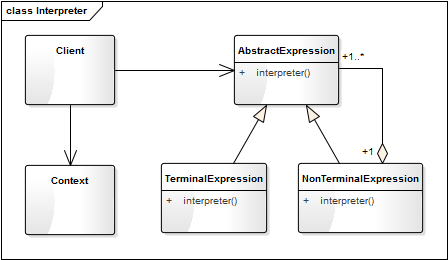AbstractExpression 解释器的抽象类，定义统一的解析方法。TerminalExpression 是终结符表达式，终结符表达式是语法中的最小单元逻辑，不可再拆分，如下面示例中的 VarExpression。

NonTerminalExpression 是非终结符表达式，方法中每一条规则对应一个非终结表达式，如下面示例中的 AddExpression 和 SubExpression。Context 是上下文环境类，包含解析器之外的一些全局信息，如下面示例中的 newExp 和 expressionMap。

应用案例

a+b+c
a+b-c

from abc import ABCMeta, abstractmethod
# 引入ABCMeta和abstractmethod来定义抽象类和抽象方法

class Expression(metaclass=ABCMeta):
"抽象表达式"

@abstractmethod
def interpreter(self, var):
pass

class VarExpression(Expression):
"变量解析器"

def __init__(self, key):
self.__key = key

def interpreter(self, var):
return var.get(self.__key)

class SymbolExpression(Expression):
"运算符解析器，运算符的抽象类"

def __init__(self, left, right):
self._left = left
self._right = right

"加法解析器"

def __init__(self, left, right):
super().__init__(left, right)

def interpreter(self, var):
return self._left.interpreter(var) + self._right.interpreter(var)

class SubExpression(SymbolExpression):
"减法解析器"

def __init__(self, left, right):
super().__init__(left, right)

def interpreter(self, var):
return self._left.interpreter(var) - self._right.interpreter(var)

# import stack
class Calculator:
"计算器类"

def __init__(self, text):
self.__expression = self.parserText(text)

def parserText(self, expText):
# 定义一个栈，处理运算的先后顺序
stack = Stack()
left = right = None # 左右表达式
idx = 0
while(idx < len(expText)):
if (expText[idx] == '+'):
left = stack.pop()
idx += 1
right = VarExpression(expText[idx])
elif(expText[idx] == '-'):
left = stack.pop()
idx += 1
right = VarExpression(expText[idx])
stack.push(SubExpression(left, right))
else:
stack.push(VarExpression(expText[idx]))
idx += 1
return stack.pop()

def run(self, var):
return self.__expression.interpreter(var)

class Stack:
"封装一个堆栈类"

def __init__(self):
self.items = []

def isEmpty(self):
return len(self.items) == 0

def push(self, item):
self.items.append(item)

def pop(self):
return self.items.pop()

def peek(self):
if not self.isEmpty():
return self.items[len(self.items) - 1]

def size(self):
return len(self.items)

def testCalculator():
# 获取表达式
expStr = input("请输入表达式：");
# 获取各参数的键值对
newExp, expressionMap = getMapValue(expStr)
calculator = Calculator(newExp)
result = calculator.run(expressionMap)
print("运算结果为:" + expStr + " = " + str(result))

def getMapValue(expStr):
preIdx = 0
expressionMap = {}
newExp = []
for i in range(0, len(expStr)):
if (expStr[i] == '+' or expStr[i] == '-'):
key = expStr[preIdx:i]
key = key.strip()  # 去除前后空字符
newExp.append(key)
newExp.append(expStr[i])
var = input("请输入参数" + key + "的值：");
var = var.strip()
expressionMap[key] = float(var)
preIdx = i + 1

# 处理最后一个参数
key = expStr[preIdx:len(expStr)]
key = key.strip()  # 去除前后空字符
newExp.append(key)
var = input("请输入参数" + key + "的值：");
var = var.strip()
expressionMap[key] = float(var)

return newExp, expressionMap